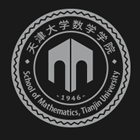﻿A Cascadic Multigrid Method for Nonsymmetric Eigenvalue Problem-天津大学数学学院

# A Cascadic Multigrid Method for Nonsymmetric Eigenvalue Problem

2019-08-26 10:47

In this talk, we proposed a cascadic multigrid method to solve nonsymmetric eigenvalue problems. Based on the multilevel correction method, the proposed method transforms a nonsymmetric eigenvalue problem solving on the finest finite element space to linear smoothing steps on a sequence of multilevel finite element spaces and some nonsymmetric eigenvalue problems solving in a very low dimensional space. Choosing the sequence of finite element spaces and the number of smoothing steps appropriately, we obtain the optimal convergence rate with the optimal scale of computational work. Some numerical examples are provided to validate the theoretical results and the efficiency of this proposed scheme.### 联系我们